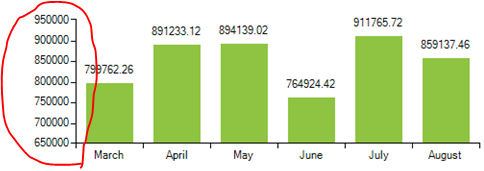# Define Y axis max and min values along with the increment in a bar chart

1. ##### Karthik1 posts
Member since:
Sep 2013

Posted 07 Oct 2013 Link to this post

I use a bar chart in my project which displays the sales data. I would like to define the max and min values and also the increment value for each step .How can this be done please help or point me out to some articles.2. ##### Dimitar2918 posts

Posted 10 Oct 2013 Link to this post

Hi Karthik,

Thank you for writing.

For example if you have the following BarSeries:
`BarSeries barSeries = ``new ``BarSeries(``"Performance"``, ``"RepresentativeName"``);`
`barSeries.Name = ``"Q1"``;`
`barSeries.DataPoints.Add(``new ``CategoricalDataPoint(500, ``"Harley"``));`
`barSeries.DataPoints.Add(``new ``CategoricalDataPoint(800, ``"White"``));`
`barSeries.DataPoints.Add(``new ``CategoricalDataPoint(143, ``"Smith"``)); `
`this``.radChartView1.Series.Add(barSeries);`

then you can set the minimum, maximum and the step as follows:
`LinearAxis verticalAxis = radChartView1.Axes.Get<LinearAxis>(1);`

`verticalAxis.Minimum = 0;`
`verticalAxis.Maximum = 1000;`
`verticalAxis.MajorStep = 100;`